# Top Data Science Interview Questions For Budding Data Scientists In 2024Ajay is a data scientist and prominent blogger in open source data...

The world of data science went through a sea change in 2015. Data scientists began threatening the role of the CIO as a company’s foremost technology influencer. With quality of data directly impacting bottom-lines, data scientists are much sought after. Add to this the popularity of Internet of Things (IoT), data science is all set to make major inroads this year.

Jobs around data science are burgeoning, bringing with it newer career opportunities and opening up growth avenues. It is highly unlikely that you wouldn’t give a data science job interview in the days to come. We at Edureka are making it a cakewalk for you by providing a list of most probable data science interview questions.

In case you have attended a data science interview in the recent past or have questions you need answers to, do paste them in the comments section and we’ll answer them ASAP.

Enroll for the Data Science course, a Post Graduate program by Edureka to elevate your career.

All the best!

## 1. What is data science? How would you say it is similar or different to business analytics and business intelligence?

• Data science is a field that deals with analysis of data. It studies the source of information, what the information represents and turning it into a valuable resource by giving insights of the data that are later used for creating strategies. It is a combination of business perspectives, computer programming and statistical techniques.
• Business analytics or simply analytics is the core of business intelligence and data science. Data science is a relatively new term used for analysis of big data and giving insights.
• Analytics generally has higher degree of business perspectives than data science which is more programming heavy. The terms are however used interchangeably.

## 2. Which package is used to do data import in R and Python? How do you do data import in SAS?

We can do data import using multiple methods:

• In R we use RODBC for RDBMS data, and data.table for fast import.
• We use jsonlite for JSON data, foreign package for other languages like SPSS
• We use data and sas7bdat package for SAS data.
• In Python we use Pandas package and the commands read_csv , read_sql for reading data. Also, we can use SQLAlchemy in Python for connecting to databases.

## 3. How do you build a custom function in Python or R?

• In R: function command

The structure of a function is given below:

myfunction <- function(arg1, arg2, … ){

statements

return(object)

}

Example:

# function example – get measures of central tendency

# and spread for a numeric vector x. The user has a

# choice of measures and whether the results are printed.

mysummary <- function(x,npar=TRUE,print=TRUE) {

if (!npar) {

center <- mean(x); spread <- sd(x)

} else {

}

if (print & !npar) {

cat(“Mean=”, center, ”
“)

} else if (print & npar) {

cat(“Median=”, center, ”
“)

}

return(result)

}

# invoking the function

set.seed(1234)

x <- rpois(500, 4)

y <- mysummary(x)

Median= 4

# y\$center is the median (4)

# y\$spread is the median absolute deviation (1.4826)

y <- mysummary(x, npar=FALSE, print=FALSE)

# no output

# y\$center is the mean (4.052)

# y\$spread is the standard deviation (2.01927)

• In Python:

def method-

Structure of the function:

def func(arg1,arg2 …):

statement 1

statement 2

return value

Example- To determine mean of a list of values.

def find_mean(given_list):

sum_values= sum(given_list)

num_values= len(given_list)

return sum_values/num_values

print find_mean([i for i in range(1,9)])

# 4

## 4. What is an RDBMS? Name some examples for RDBMS? What is CRUD?

• A relational database management system (RDBMS) is a database management system that is based on a relational model. The relational model uses the basic concept of a relation or table. RDBMS is the basis for SQL, and for database systems like MS SQL Server, IBM DB2, Oracle, MySQL, and Microsoft Access.
• In computer programming, create, read, update and delete (as an acronym CRUD or possibly a backronym) (Sometimes called SCRUD with an “S” for Search) are the four basic functions of persistent storage.

## 5. Define a SQL query? What is the difference between SELECT and UPDATE Query? How do you use SQL in SAS, Python, R languages?

• Structured query language (SQL) is a programming language designed for getting information from and updating a database. They are used for managing data in relational database management system (RDBMS). Queries take the form of a command language that lets you select, insert, update, find out the location of data, and so forth. There is also a programming interface.
• The UPDATE query is used to update existing records in a table. The SELECT query is used to select data from a database. The result is stored in a result table, called the result-set.
• We use PROC SQL within SAS, sqldf from within R and pandasql from within Python for using SQL sytax. SHOW EXAMPLES
• Python-

SQLAlchemy Library: This allows you to execute raw SQL queries on tables in database present in MySQL-server from python. These also exists SQLAlchemy Expression Language which represents relational database structures and expressions using Python constructs. The expression language improves the maintainability of the code by hiding the SQL language and thus disallowing a mix of Python code and SQL code.

import sqlalchemy

engine =

from sqlalchemy import text

with engine.connect() as con:

rs = con.execute(text(‘SELECT * FROM BigDiamonds limit 1’))

print rs.keys()

print rs.fetchall()

[u’Unnamed’, u’carat’, u’cut’, u’color’, u’clarity’, u’tabl’, u’depth’, u’cert’, u’measurements’, u’price’, u’x’, u’y’, u’z’] [(1L, 0.25, ‘V.Good’, ‘K’, ‘I1’, 59.0, 63.7, ‘GIA’, ‘3.96 x 3.95 x 2.52’, 0.0, 3.96, 3.95, 2.52)]

PandaSQL: allows you to query pandas DataFrames using SQL syntax. It works similarly to sqldf in R. For people new to Python or pandas it provides an easy functionality.

from pandasql import sqldf

pysqldf(“SELECT * FROM mycars LIMIT 1;”)

 brand mpg cyl disp hp drat wt qsec vs am gear carb 0 Mazda RX4 21.0 6 160.0 110 3.90 2.620 16.46 0 1 4
• R-

library(sqldf)

sqldf(“select medv,rm from Boston limit 1”)

## medv rm

## 1 34.7 7.185

## 6. What is an API ? What are APIs used for?

API, an abbreviation of application program interface, is a set of routines, protocols, and tools for building software applications. The API specifies how software components should interact and APIs are used when programming graphical user interface (GUI) components.

A good API makes it easier to develop a program by providing all the building blocks. There are many types of APIs for operating systems, applications or for websites.

## 7. What is NoSQL? Name some examples of NoSQL databases. What is a key value store? What is column storage? What is a document database?

NoSQL refers to the non-relational database. It is used for storage and retrieval of data that is modeled in means other than the tabular relations used in relational databases. Some examples of NoSQL databases are:

• MongoDB
• CouchDB
• BigTable
• Redis
• RavenDb

A key-value store, or key-value database, is a data storage paradigm designed for storing, retrieving, and managing associative arrays, a data structure more commonly known today as a dictionary or hash. Dictionaries contain a collection of objects, or records, which in turn have many different fields within them, each containing data. These records are stored and retrieved using a key that uniquely identifies the record, and is used to quickly find the data within the database.

A columnar database is a database management system (DBMS) that stores data in columns instead of rows. The goal of a columnar database is to efficiently write and read data to and from hard disk storage in order to speed up the time it takes to return a query.

Here is an example of a simple database table with 4 columns and 3 rows.

ID Last First Bonus

1 Doe John 8000

2 Smith Jane 4000

3 Beck Sam 1000

## 8. What is a data warehouse?

A data warehouse is a large store of data collected from a wide range of sources within an enterprise. It is also known as the central repository of integrated data. The repository maybe physical or logical.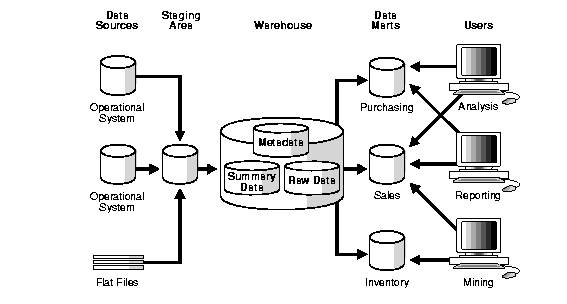## 9. What is JSON and What is XML?

JSON is an abbreviation for JavaScript Object Notation. It is a primary data format that uses human-readable text to transfer data objects consisting of data interpretation language. Although originally derived from the JavaScript scripting language, JSON is a language-independent data format. Code for generating JSON data is readily available in many programming languages.
XML is an abbreviation for Extensible Markup Language. It defines a set of rules that is used for encoding documents in a human and machine readable format. The design goals of XML emphasize simplicity, generality and usability across the Internet. It is a textual data format with strong support for different human languages. It is widely used for the representation of arbitrary data structures such as those used in web services.

## 10. Name some kinds of graphs and explain how you would build them in Python or R.

Basic graphs in R: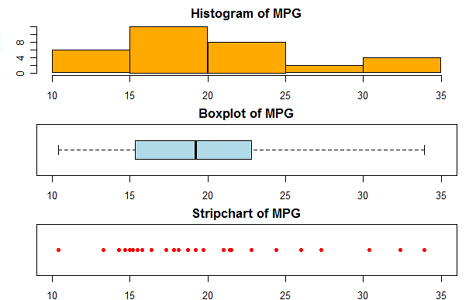Creating a Graph:

In R, graphs are typically created interactively.

# Creating a Graph

attach(mtcars)

plot(wt, mpg)

abline(lm(mpg~wt))

title(“Regression of MPG on Weight”)

The plot( ) function opens a graph window and plots weight vs. miles per gallon.

The next line of code adds a regression line to this graph. The final line adds a title.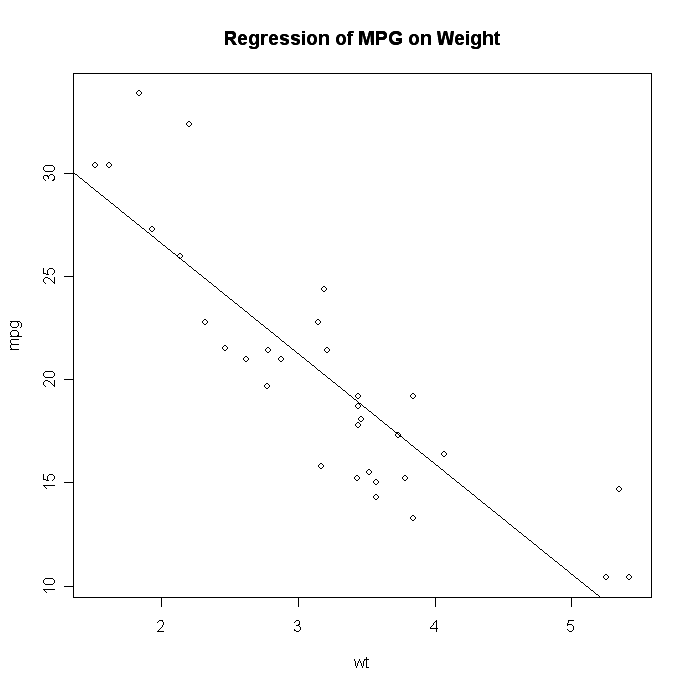## 11. How do you check for data quality?

Data quality is an assessment of data’s fitness to serve its purpose in a given context. Different aspects of data quality include:

• Accuracy
• Completeness
• Update status
• Relevance
• Consistency across data sources
• Reliability
• Appropriate presentation
• Accessibility

Maintaining data quality requires going through the data in different intervals and scrubbing it. This involves updating it, standardizing it, and removing duplicates to create a single view of the data, even if it is stored in multiple systems.

## 12. What is an outlier? How do you treat outlier data?

An outlier is an unusual observation that lie at an abnormal distance from the other values in a random sample of the data. Before abnormal observations can be singled out, it is necessary to characterize normal observations. Outliers can be of two types:

1. Univariate – They are present in a single variable data
2. Multivariate – They are present in an n-dimensional space

Outliers should be investigated carefully. Often they contain valuable information about the process under investigation or the data gathering and recording process. Before considering the possible elimination of these points from the data, one should try to understand why they appeared and whether it is likely similar values will continue to appear. Of course, outliers are often bad data points.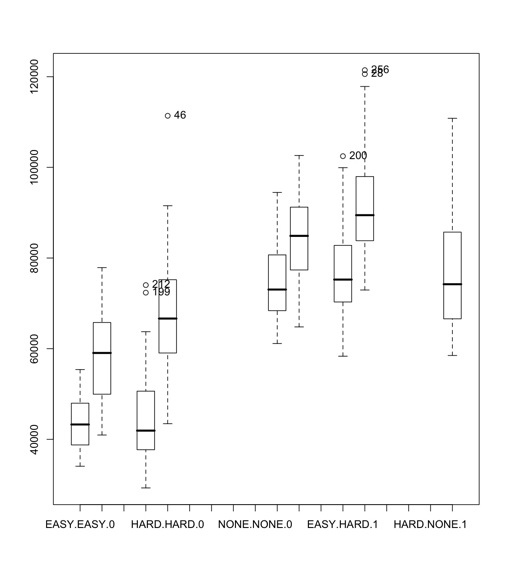Methods to detect outliers:

• We can detect outliers by visualization, various methods include Box-plot, Histogram, Scatter Plot.
• Any value, which is beyond the range of -1.5 x IQR to 1.5 x IQR
• Use capping methods. Any value which out of range of 5th and 95th percentile can be considered as outlier
• Data points, three or more standard deviation away from mean are considered outlier
• Outlier detection is merely a special case of the examination of data for influential data points and it also depends on the business understanding
• Bivariate and multivariate outliers are typically measured using either an index of influence or leverage, or distance. Popular indices such as Mahalanobis’ distance and Cook’s D are frequently used to detect outliers.
• In SAS, we can use PROC Univariate, PROC SGPLOT. To identify outliers and influential observation, we also look at statistical measure like STUDENT, COOKD, RSTUDENT and others.

Removing outliers:

• Deleting observations – We remove the outlier values
• Transforming values – We can transform the values for eg. Taking a natural log to reduce the variation.
• Imputing – We can impute the data using mean, median, mode imputation methods.
• Treat separately – If there are significant number of outliers, we should treat them separately in the statistical model.

## 13. What is missing value imputation? How do you handle missing values in Python or R?

Imputation is the process of replacing missing data with substitute values.

IN R

Missing values are represented in R by the NA symbol. NA is a special value whose properties are different from other values. NA is one of the very few reserved words in R: you cannot give anything this name. Here are some examples of operations that produce NA’s.

> var (8) # Variance of one number

 NA

> as.numeric (c(“1”, “2”, “three”, “4”)) # Illegal conversion

 1 2 NA 4

Operations on missing values:

Almost every operation performed on an NA produces an NA. For example:

> x <- c(1, 2, NA, 4) # Set up a numeric vector

> x # There’s an NA in there

 1 2 NA 4

> x + 1 # NA + 1 = NA

Excluding missing values:

Math functions generally have a way to exclude missing values in their calculations. mean(), median(), colSums(), var(), sd(), min() and max() all take the na.rm argument. When this is TRUE, missing values are omitted. The default is FALSE, meaning that each of these functions returns NA if any input number is NA. Note that cor() and its relatives don’t work that way: with those you need to supply the use= argument. This is to permit more complicated handling of missing values than simply omitting them.

R’s modeling functions accept an na.action argument that tells the function what to do when it encounters an NA. The filter functions are:

• na.fail: Stop if any missing values are encountered
• na.omit: Drop out any rows with missing values anywhere in them and forgets them forever
• na.exclude: Drop out rows with missing values, but keeps track of where they were (so that when you make predictions, for example, you end up with a vector whose length is that of the original response.)
• na.pass: Take no action.

A couple of other packages supply more alternatives:

• na.tree.replace (library (tree): For discrete variables, adds a new category called “NA” to replace the missing values
• na.gam.replace (library gam): Operates on discrete variables like na.tree.replace(); for numerics, NAs are replaced by the mean of the non-missing entries.

Python:

Missing values in pandas are represented by NaN or None. They can be detected using isnull() and notnull() functions.

Operations on missing values

For all math functions sum(), mean(), max(), min() NA (missing) values will be treated as zero. If the data are all NA, the result will be NA.

df[“one”]

one

a NaN

c NaN

e 0.294633

f -0.685597

h NaN

df[“one”].sum()

-0.39096437337883205

Cleaning/filling missing values

• fillna- can fill in NA values with non-null data
• dropna – to remove axis containing missing values.

Imputing missing data:

Imputer is a transformer algorithm in scikitlearn library in python used to complete missing values to determine the best value for the missing data. Example:-

import pandas as pd

import numpy as np

from sklearn.preprocessing import Imputer

s = pd.Series([1, 2, 3, np.NaN, 5, 6, None])

imp = Imputer(missing_values=‘NaN’,

strategy=‘mean’, axis=0)

imp.fit([1, 2, 3, 4, 5, 6, 7])

x = pd.Series(imp.transform(s).tolist())

print x

output-

0 1

1 2

2 3

3 4

4 5

5 6

6 7

dtype: float64

## 14. Why do you need a for loop? How do you do for loops in Python and R?

We use the ‘for’ loop if we need to do the same task a specific number of times.

In R, it looks like this:

for (counter in vector) {commands}

We will set up a loop to square every element of the dataset, foo, which contains the odd integers from 1 to 100 (keep in mind that vectorizing would be faster for a trivial example – see below):

foo = seq(1, 100, by=2)

foo.squared = NULL

for (i in 1:50 ) {

foo.squared[i] = foo[i]^2

}

If the creation of a new vector is the goal, first we have to set up a vector to store things in prior to running the loop. This is the foo.squared = NULL part.

Next, the real for-loop begins. This code says we’ll loop 50 times(1:50). The counter we set up is ‘i’ (but we can put whatever variable name we want there). For our new vector foo.squared, the ith element will equal the number of loops that we are on (for the first loop, i=1; second loop, i=2).

## 15. What is advantage of using apply family of functions in R? How do you use lambda in Python?

The apply function allows us to make entry-by-entry changes to data frames and matrices.

The usage in R is as follows:

apply(X, MARGIN, FUN, …)

where:

X is an array or matrix;

MARGIN is a variable that determines whether the function is applied over rows (MARGIN=1), columns (MARGIN=2), or both (MARGIN=c(1,2));

FUN is the function to be applied.

If MARGIN=1, the function accepts each row of X as a vector argument, and returns a vector of the results. Similarly, if MARGIN=2 the function acts on the columns of X. Most impressively, when MARGIN=c(1,2) the function is applied to every entry of X.

With the apply function we can edit every entry of a data frame with a single line command. No auto-filling, no wasted CPU cycles.

Lambda-

afunc=lambda a: func_on_a

You can then use lambda with map, reduce and filter functions based on requirement. Lambda applies the function on elements one at a time.

## 16. What packages are used for data mining in Python and R?

Python:

• Scikit-learn – Machine learning library, built on top of NumPy, SciPy and matplotlib.
• NumPy and SciPy– for providing mathematical functionality like Matlab.
• Matplotlib- Visualization library, provides plots like in Matlab.
• NLTK– Natural Language Processing library. Extensively used fot textminng.
• Orange– Provides visualization and machine learning features. Also provies association rule learning.
• Pandas- Inspired from R. Provides functionality of working on dataframe.

R:

• data.table- provides fast reading of large files
• rpart and caret- for machine learning models.
• Arules- for associaltion rule learning.
• GGplot- provides varios data visualization plots.
• tm- to perform text mining.
• Forecast- provides functions for time series analysis

## 17. What is machine learning? What is the difference between supervised and unsupervised methods?

Machine learning studies computer algorithms for learning to do stuff. There are many examples of machine learning problems. For e.g.:

• optical character recognition: categorize images of handwritten characters by the letters represented
• face detection: find faces in images (or indicate if a face is present)
• spam filtering: identify email messages as spam or non-spam
• topic spotting: categorize news articles (say) as to whether they are about politics, sports, entertainment, etc.
• spoken language understanding: within the context of a limited domain, determine the meaning of something uttered by a speaker to the extent that it can be classified into one of a fixed set of categories
• medical diagnosis: diagnose a patient as a sufferer or non-sufferer of some disease
• customer segmentation: predict, for instance, which customers will respond to a particular promotion
• fraud detection: identify credit card transactions (for instance) which may be fraudulent in nature
• weather prediction: predict, for instance, whether or not it will rain tomorrow

Supervised learning is the type of learning that takes place when the training instances are labelled with the correct result, which gives feedback about how learning is progressing. Supervised learning is fairly common in classification problems because the goal is often to get the computer to learn a classification system that we have created. Digit recognition is a common example of classification learning.

In unsupervised learning, there are no pre-determined categorizations. There are two approaches to unsupervised learning:

1. The first approach is to teach the agent not by giving explicit categorizations, but by using some sort of reward system to indicate success. This approach nicely generalizes to the real world, where agents might be rewarded for doing certain actions and punished for doing others.
2. A second type of unsupervised learning is called clustering. In this type of learning, the goal is not to maximize a utility function, but simply to find similarities in the training data. The assumption is often that the clusters discovered will match reasonably well with an intuitive classification. For instance, clustering individuals based on demographics might result in a clustering of the wealthy in one group and the poor in another.

## 18. What is random forests and how is it different from decision trees?

Random forests involves building several decision trees based on sampling features and then making predictions based on majority voting among trees for classification problems or average for regression problems. This solves the problem of overfitting in Decision Trees.

Algorithm:-

Repeat K times:

• Draw a bootstrap sample from the dataset.
• Train a Decision Tree by selecting m features from available p features.
• Measure out of bag error. Evaluate against the samples which were not selected in bootstrap.

Make a prediction by majority voting among K trees

Random Forests are more difficult to interpret than single decision trees, so understanding variable importance helps.

Random forests are easy to parallelize, trees can be built independently. Handles NbigP-Problems naturally since a subset of attributes are selected by importance.

## 19. What is logistic and linear regression? How do you treat multicollinearity and heteroscedasticity in regression? Name some packages in R and Python for building regression models.

Linear Regression:

Here we try to predict results within a continuous output. Hypothesis-

htheta(x)= theta0 + theta1x1 + theta2x2 …

Logistic Regression:

Here we try to map input variables into discrete categories. Used to solve classification problems. Hypothesis-

htheta(x)= g(thetaT x)

g(z)= 1/(1 + exp(-z))

It is known as logistic or sigmoid function.

htheta(x) in logistic regression is the measure of probability the sample belongs to a particular class. 0<=htheta(x)<=1

Multicollinearity refers to predictors that are correlated with other predictors. Multicollinearity occurs when your model includes multiple factors that are correlated not just to your response variable, but also to each other. In other words, it results when you have factors that are a bit redundant.

To measure multicollinearity variance inflation factor (VIF) is used, which assesses how much the variance of an estimated regression coefficient increases if your predictors are correlated. If no factors are correlated, the VIFs will all be 1. A VIF between 5 and 10 indicates high correlation that may be problematic. And if the VIF goes above 10, you can assume that the regression coefficients are poorly estimated due to multicollinearity.

To deal with Multicollinearity Try any one of the following methods:-

• Remove highly correlated predictors from the model. If there exists two or more factors with high VIF, remove one of them since they supply redundant information.
• Use Partial Least Squares Regression (PLS) or Principal Components Analysis, regression.

Heteroscedasticity:

A scatterplot of variables often create a cone-like shape, as the scatter (or variability) of the dependent variable widens or narrows as the value of the independent variable increases. This is known as heteroscedasticity. More formally it refers to the circumstance in which the variability of a variable is unequal across the range of values of a second variable that predicts it.

Packages for regression models in –

Python- StatsModels or Generalized Linear Models in Scikitlearn.

R – glm and lm functions.

## 20. What is linear optimization? Where is it used? What is the travelling salesman problem? How do you use Goal Seek in Excel?

Linear optimization or Linear Programming (LP) involves minimizing or maximizing an objective function subject to bounds, linear equality, and inequality constraints. Example problems include design optimization in engineering, profit maximization in manufacturing, portfolio optimization in finance, and scheduling in energy and transportation.

The following algorithms are commonly used to solve linear programming problems:

• Interior point: Uses a primal-dual predictor-corrector algorithm and is especially useful for large-scale problems that have structure or can be defined using sparse matrices.
• Active-set: Minimizes the objective at each iteration over the active set (a subset of the constraints that are locally active) until it reaches a solution.
• Simplex: Uses a systematic procedure for generating and testing candidate vertex solutions to a linear program. The simplex algorithm is the most widely used algorithm for linear programming.

Travelling Salesman Problem belongs to the class of np-complete problems. TSP is a special case of the travelling purchaser problem and the Vehicle routing problem. It is used as a benchmark for many optimization methods. It is a problem in graph theory requiring the most efficient i.e. least squared distance a salesman can take through n cities.

## 21. What is CART and CHAID? How is bagging different from boosting?

CART:

• Classification And Regression Tree (CART) analysis is an umbrella term used to refer to Classification Tree analysis in which the predicted outcome is the class to which the data belongs. and Regression Tree analysis in which the predicted outcome can be considered a real number.
• Splits in Tree are made by variables that best differentiate the target variable.
• Each node can be split into two child nodes.
• Stopping rule governs the size of the tree.

CHAID:

• Chi Square Automatic Interaction Detection.
• Performs multi-level splits whereas CART uses binary splits.
• Well suited for large data sets.
• Commonly used for market segmentation studies.

Bagging:

• Draw N bootstrap samples.
• Retrain the model on each Sample.
• Average the results
– Regression – Averaging
– Classification – Majority Voting
• Works great for overfit models
– Decreases variance without changing bias.
– Doesn’t help much with underfit/high bias models.
• Insensitive to training data.

Boosting:

• Instead of selecting data points randomly with bootstrap favor the mis-classified points by adjusting the weights down for correctly classified examples.
• Here sequentiality is present so difficult to apply in case of large data.

## 22. What is clustering? What is the difference between kmeans clustering and hierarchical clustering?

Cluster is a group of objects that belongs to the same class. Clustering is the process of making a group of abstract objects into classes of similar objects.

Let us see why clustering is required in data analysis:

• Scalability − We need highly scalable clustering algorithms to deal with large databases.
• Ability to deal with different kinds of attributes − Algorithms should be capable of being applied to any kind of data such as interval-based (numerical) data, categorical, and binary data.
• Discovery of clusters with attribute shape − The clustering algorithm should be capable of detecting clusters of arbitrary shape. They should not be bounded to only distance measures that tend to find spherical cluster of small sizes.
• High dimensionality − The clustering algorithm should not only be able to handle low-dimensional data but also the high dimensional space.
• Ability to deal with noisy data − Databases contain noisy, missing or erroneous data. Some algorithms are sensitive to such data and may lead to poor quality clusters.
• Interpretability − The clustering results should be interpret-able, comprehensible, and usable.

K-MEANS clustering:

K-means clustering is a well known partitioning method. In this method objects are classified as belonging to one of K-groups. The results of partitioning method are a set of K clusters, each object of data set belonging to one cluster. In each cluster there may be a centroid or a cluster representative. In the case where we consider real-valued data, the arithmetic mean of the attribute vectors for all objects within a cluster provides an appropriate representative; alternative types of centroid may be required in other cases.

Example: A cluster of documents can be represented by a list of those keywords that occur in some minimum number of documents within a cluster. If the number of the clusters is large, the centroids can be further clustered to produce hierarchy within a dataset. K-means is a data mining algorithm which performs clustering of the data samples. In order to cluster the database, K-means algorithm uses an iterative approach.

R code

# Determine number of clusters

wss <- (nrow(mydata)-1)*sum(apply(mydata,2,var))

for (i in 2:15) wss[i] <- sum(kmeans(mydata,

centers=i)\$withinss)

plot(1:15, wss, type=”b”, xlab=”Number of Clusters”,

ylab=”Within groups sum of squares”)

# K-Means Cluster Analysis

fit <- kmeans(mydata, 5) # 5 cluster solution

# get cluster means

aggregate(mydata,by=list(fit\$cluster),FUN=mean)

# append cluster assignment

mydata <- data.frame(mydata, fit\$cluster)

A robust version of K-means based on mediods can be invoked by using pam( ) instead of kmeans( ). The function pamk( ) in the fpc package is a wrapper for pam that also prints the suggested number of clusters based on optimum average silhouette width.

Hierarchical Clustering:

This method creates a hierarchical decomposition of the given set of data objects. We can classify hierarchical methods on the basis of how the hierarchical decomposition is formed. There are two approaches here:

1. Agglomerative Approach
2. Divisive Approach

Agglomerative Approach:

This approach is also known as the bottom-up approach. In this, we start with each object forming a separate group. It keeps on merging the objects or groups that are close to one another. It keeps on doing so until all of the groups are merged into one or until the termination condition holds.

Divisive Approach:

This approach is also known as the top-down approach. In this, we start with all of the objects in the same cluster. In the continuous iteration, a cluster is split up into smaller clusters. It is down until each object in one cluster or the termination condition holds. This method is rigid, i.e., once a merging or splitting is done, it can never be undone.

R code

Cars example

# The mtcars data set is built into R:

help(mtcars)

# We will focus on the variables that are continuous in nature rather than discrete:

cars.data <- mtcars[,c(1,3,4,5,6,7)]

# Standardizing by dividing through by the sample range of each variable

samp.range <- function(x){

myrange <- diff(range(x))

return(myrange)

}

my.ranges <- apply(cars.data,2,samp.range)

cars.std <- sweep(cars.data,2,my.ranges,FUN=”/”)

# Getting distance matrix:

dist.cars <- dist(cars.std)

# Plotting the single linkage dendrogram:

windows() # opening new window while keeping previous one open

# Plotting the complete linkage dendrogram:

windows() # opening new window while keeping previous one open

# Plotting the average linkage dendrogram:

# Average Linkage dendrogram seems to indicate two major clusters,

# Single Linkage dendrogram may indicate three.

cut.3 # printing the “clustering vector”

cars.3.clust <- lapply(1:3, function(nc) row.names(cars.data)[cut.3==nc])

cars.3.clust # printing the clusters in terms of the car names

# Cluster 1 seems to be mostly compact cars, Cluster 2 is sports cars, Cluster 3 is large Luxury sedans

## 23. What is churn? How would it help predict and control churn for a customer?

Customer churn, also known as customer attrition, customer turnover, or customer defection, is the loss of clients or customers.

Banks, telephone service companies, internet service providers, pay TV companies, insurance firms, and alarm monitoring services, often use customer churn analysis and customer churn rates as one of their key business metrics because the cost of retaining an existing customer is far less than acquiring a new one. Companies from these sectors often have customer service branches which attempt to win back defecting clients, because recovered long-term customers can be worth much more to a company than newly recruited clients.

The statistical methods, which have been applied for decades in medicine and engineering, come in handy any time we are interested in understanding how long something (customers, patients, car parts) survives and what actions can help it survive longer.

Understanding through a problem:

Let’s frame the survival analysis idea using an illustrative example. We’ll be using this example (and associated dummy datasets).

Suppose you work at NetLixx, an online startup which maintains a library of guitar tabs for popular rock hits. Guitar enthusiasts can pay \$5 a month for a subscription to your library, which lets them display the tabs on their computer, tablet, or phone while they rock out. After a year of hard work, you’ve got a working site, an extensive database of music, and a couple thousand customers.

But you’re also concerned. You’ve had a lot of people sign up for your service, but many seem to be quitting in only a couple of months. You want to know how long your customers are likely to stay with you, and whether customers with a certain demographic profile tend to churn more slowly.

You could, of course, try some basic statistics, but you’ll quickly find yourself stuck between a rock and a hard place.

• The rock – You need to follow customers for a few months (ideally a year) to get any meaningful information, especially since this is a monthly subscription.
• The hard place – Your business has only been around for a year! A good majority of your customers don’t have five or six months (let alone a year) of data to follow up on.

So, what’s an analyst to do? Well, luckily, this is a case where you can have your cake and eat it too. How? With Kaplan-Meier estimators, for starters!

Kaplan-Meier Estimators:

Kaplan-Meier estimators predict survival probabilities over a given period of time for “right-censored” data. “Right-censored” just means that some of the observations in the data weren’t observed for as long as the period the researcher is interested in analyzing. (For example, we want to look at a year of churn, but some of our customers signed up a month ago). Kaplan-Meier estimators reliably incorporate all available data at each individual time interval to estimate how many observations are still “surviving” at that time.

To do simple survival analysis using these estimators, all you need is a table of customers with a binary value indicating whether they’ve churned, and a “follow-up time.” The follow-up time can take on one of two values. If the customer churned, it’s the number of days (or weeks, months, whatever) between the day they subscribed and the day they unsubscribed. Otherwise, it’s just the number of days between the day they subscribed and today (or the day the data was pulled).

For this , we’ll be using a simple CSV file of NetLixx data as an example. (Download the NetLixx data here.) The data includes follow-up time, a churn binary, and a gender indicator. The first few observations are displayed below. Note how the second customer has a follow-up time of 360, while the third has a follow-up time of 8, even though neither have churned. This means customer 2 signed up 360 days ago, but customer 3 signed up only 8 days ago. Neither have left us yet!

 Female Time Churned 0 273 0 0 360 0 0 8 0 0 211 0

Let’s plot some data! For this analysis, we’ll be using R and the “survival” package, since both are free tools, and they work great for basic survival analysis.

Here’s some simple R code that uses the survival package to fit Kaplan-Meier estimators and plot a simple survival curve. (If you’re new to this… don’t forget to install the package with ‘install.packages(“survival”).’)

# Use the survival library

library(survival)

# Read in the NetLixx data… yes, I use Windows.

# Create a “survival object” for each observation, using time and churn data.

net_lixx\$survival <- Surv(net_lixx\$time, net_lixx\$churned == 1)

# Fit a basic survival curve using the data

fit <- survfit(survival ~ 1, data = net_lixx)

# Plot the survival curve and add a title!

plot(fit, lty = 1, mark.time = FALSE, ylim=c(.75,1), xlab = ‘Days since Subscribing’, ylab = ‘Percent Surviving’)

title(main = ‘NetLixx Survival Curve’)

And here’s the resulting plot! That line in the middle represents the best estimate of the percent of customers surviving at each time interval. The dashed lines represent a 95% confidence interval. The confidence interval spreads out as we get closer to 365 days, since we have less and less customers with that much data to work with.

Looking at this graph, we know that we can expect 75% of customers (give or take) to make it through their first year with us… not bad!

So, there you have it, the basic Kaplan-Meier estimator.Looking for Trend:

Of course, knowing how fast our customers churn is all well and good, but what we’re really interested in is understanding and analyzing churn. We want to know what makes a customer more likely to churn, and what causes them to stick around.

One easy way to do that is to create different Kaplan-Meier survival curves for each subset of subscribers you want to look at. The statistical significance of the differences can be tested in many ways, including the Log-Rank test. The Log-Rank test simply evaluates whether the underlying population survival curves for the two sampled groups are likely to be the same. The p-value is essentially the probability that the curves are the same, so statistical significance (I’ll use p < .05) is good!

R code

# Run basic setup again

library(survival)

net_lixx\$survival <- Surv(net_lixx\$time, net_lixx\$churned == 1)

# Fit survival curves based on the female binary

fit <- survfit(survival ~ female, data = net_lixx)

plot(fit, lty = 1:2, mark.time = FALSE, ylim=c(.75,1), xlab = ‘Days since Subscribing’, ylab = ‘Percent Surviving’)

legend(20, .8, c(‘Male’, ‘Female’), lty=1:2, bty = ‘n’, ncol = 2)

title(main = “NetLixx Survival Curves by Gender”)

# And run a log-rank test

survdiff(survival ~ female, data = net_lixx)

## 24. What is market basket analysis? How would you do it in R and Python?

Market basket analysis is the study of items that are purchased or grouped together in a single transaction or multiple, sequential transactions. Understanding the relationships and the strength of those relationships is valuable information that can be used to make recommendations, cross-sell, up-sell, offer coupons, etc.

The analysis reveals patterns such as that of the well-known study which found an association between purchases of diapers and beer.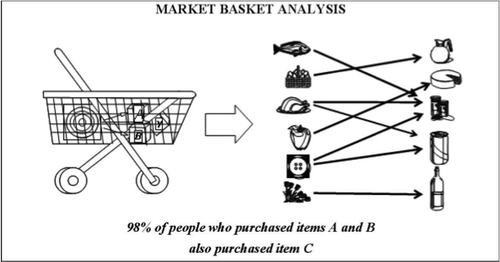In a market basket analysis the transactions are analysed to identify rules of association. For example, one rule could be: {pencil, paper} => {rubber}. This means that if a customer has a transaction that contains a pencil and paper, then they are likely to be interested in also buying a rubber.

Before acting on a rule, a retailer needs to know whether there is sufficient evidence to suggest that it will result in a beneficial outcome. We therefore measure the strength of a rule by calculating the following three metrics (note other metrics are available, but these are the three most commonly used):

1. Support: the percentage of transactions that contain all of the items in an item set (e.g., pencil, paper and rubber). The higher the support the more frequently the item set occurs. Rules with a high support are preferred since they are likely to be applicable to a large number of future transactions.
2. Confidence: the probability that a transaction that contains the items on the left hand side of the rule (in our example, pencil and paper) also contains the item on the right hand side (a rubber). The higher the confidence, the greater the likelihood that the item on the right hand side will be purchased or, in other words, the greater the return rate we can expect for a given rule.
3. Lift: the probability of all of the items in a rule occurring together (otherwise known as the support) divided by the product of the probabilities of the items on the left and right hand side occurring as if there was no association between them. For example, if pencil, paper and rubber occurred together in 2.5% of all transactions, pencil and paper in 10% of transactions and rubber in 8% of transactions, then the lift would be: 0.025/(0.1*0.08) = 3.125. A lift of more than 1 suggests that the presence of pencil and paper increases the probability that a rubber will also occur in the transaction. Overall, lift summarizes the strength of association between the products on the left and right hand side of the rule; the larger the lift the greater the link between the two products.

·To perform a Market Basket Analysis and identify potential rules, a data mining algorithm called the ‘Apriori algorithm’ is commonly used, which works in two steps:

1. Systematically identify item sets that occur frequently in the data set with a support greater than a pre-specified threshold.
2. Calculate the confidence of all possible rules given the frequent item sets and keep only those with a confidence greater than a pre-specified threshold.

The thresholds at which to set the support and confidence are user-specified and are likely to vary between transaction data sets. R does have default values, but we recommend you experiment with these to see how they affect the number of rules returned.

Performing Market Basket Analysis in R:

We are using arulespackage for performing a market basket analysis.

We use a data set of grocery sales that contains 9,835 individual transactions with 169 items. The first thing we do is have a look at the items in the transactions and, in particular, plot the relative frequency of the 25 most frequent items in Figure 1. This is equivalent to the support of these items where each item set contains only the single item. This bar plot illustrates the groceries that are frequently bought at this store, and it is notable that the support of even the most frequent items is relatively low (for example, the most frequent item occurs in only around 2.5% of transactions). We use these insights to inform the minimum threshold when running the Apriori algorithm; for example, we know that in order for the algorithm to return a reasonable number of rules we’ll need to set the support threshold at well below 0.025.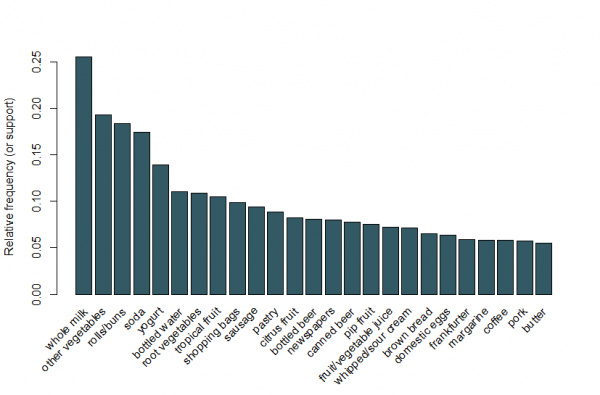Figure 1: A bar plot of the support of the 25 most frequent items bought.

By setting a support threshold of 0.001 and confidence of 0.5, we can run the Apriori algorithm and obtain a set of 5,668 results. These threshold values are chosen so that the number of rules returned is high, but this number would reduce if we increased either threshold. We would recommend experimenting with these thresholds to obtain the most appropriate values. Whilst there are too many rules to be able to look at them all individually, we can look at the five rules with the largest lift:

 Rule Product Support Confidence Lift {instant food products, soda} =>{hamburger meat} 0.001 0.632 19.000 {soda, popcorn} =>{salty snacks} 0.001 0.632 16.698 {flour, baking powder} =>{sugar} 0.001 0.556 16.408 {ham, processed cheese} =>{white bread} 0.002 0.633 15.045 {whole milk, instant food products} =>{hamburger meat} 0.002 0.500 15.038

These rules seem to make intuitive sense. For example, the first rule might represent the sort of items purchased for a BBQ, the second for a movie night and the third for baking.

Rather than using the thresholds to reduce the rules down to a smaller set, it is usual for a larger set of rules to be returned so that there is a greater chance of generating relevant rules. Alternatively, we can use visualisation techniques to inspect the set of rules returned and identify those that are likely to be useful.

Using the arulesViz package, we plot the rules by confidence, support and lift in Figure 2. This plot illustrates the relationship between the different metrics. It has been shown that the optimal rules are those that lie on what’s known as the “support-confidence boundary”. Essentially, these are the rules that lie on the right hand border of the plot where either support, confidence or both are maximised. The plot function in the arulesViz package has a useful interactive function that allows us to select individual rules (by clicking on the associated data point), which means the rules on the border can be easily identified.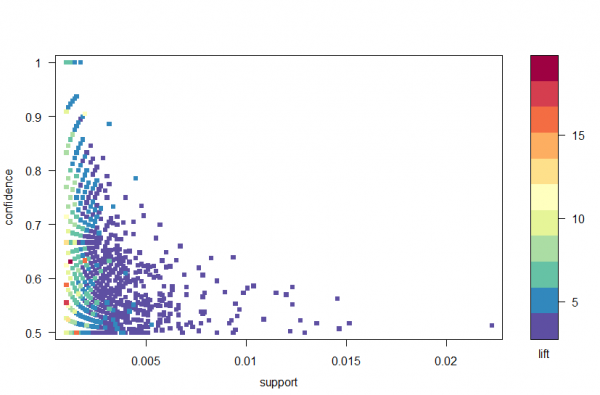Figure 2: A scatter plot of the confidence, support and lift metrics.

There are lots of other plots available to visualize the rules, but one other figure that we would recommend exploring is the graph-based visualization (see Figure 3) of the top ten rules in terms of lift. In this graph the items grouped around a circle represent an item set and the arrows indicate the relationship in rules. For example, one rule is that the purchase of sugar is associated with purchases of flour and baking powder. The size of the circle represents the level of confidence associated with the rule and the color the level of lift (the larger the circle and the darker the grey the better).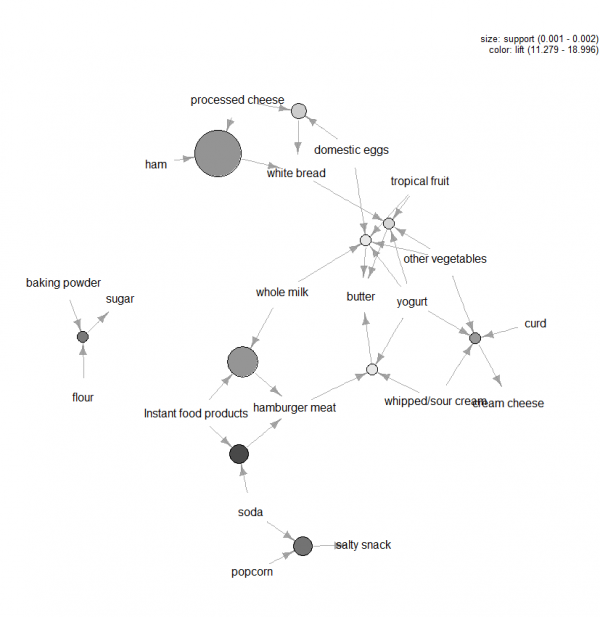Figure 3: Graph-based visualization for the top ten rules in terms of lift.

There are many tools that can be applied when carrying out a market basket analysis and the trickiest aspects to the analysis are setting the confidence and support thresholds in the Apriori algorithm and identifying which rules are worth pursuing.

Typically the latter is done by measuring the rules in terms of metrics that summarize how interesting they are, using visualization techniques and also more formal multivariate statistics. Ultimately the key to market basket analysis is to extract value from the transaction data by building up an understanding of the needs of the consumers.

R code

library(“arules”)

library(“arulesViz”)

data(“Groceries”)

summary(Groceries)

#Look at data:

inspect(Groceries)

LIST(Groceries)

#Calculate rules using apriori algorithm and specifying support and confidence thresholds:

rules = apriori(Groceries, parameter=list(support=0.001, confidence=0.5))

#Inspect the top 5 rules in terms of lift:

#Plot a frequency plot:

itemFrequencyPlot(Groceries, topN = 25)

#Scatter plot of rules:

library(“RColorBrewer”)

plot(rules,control=list(col=brewer.pal(11,”Spectral”)),main=””)

#Rules with high lift typically have low support.

#The most interesting rules reside on the support/confidence border which can be clearly seen in this plot.

#Plot graph-based visualisation:

plot(subrules2, method=”graph”,control=list(type=”items”,main=””))

## 25. What is association analysis? Where is it used?

Association analysis uses a set of transactions to discover rules that indicate the likely occurrence of an item based on the occurrences of other items in the transaction. The technique of association rules is widely used for retail basket analysis. It can also be used for classification by using rules with class labels on the right-hand side. It is even used for outlier detection with rules indicating infrequent/abnormal association.

Association analysis also helps us to identify cross-selling opportunities, for example: we can use the rules resulting from the analysis to place associated products together in a catalog, in the supermarket, or in the Web shop, or apply them when targeting a marketing campaign for product B at customers who have already purchased product A

Association analysis determines these rules by using historic data to train the model. We can display and export the determined association rules.

## 26. What is the central limit theorem? How is a normal distribution different from chi square distribution?

Central limit theorem states that the distribution of an average will tend to be Normal as the sample size increases, regardless of the distribution from which the average is taken except when the moments of the parent distribution do not exist. All practical distributions in statistical engineering have defined moments, and thus the CLT applies.

Chi square distribution uses standard normal variates which are a part of normal distribution. In statistical terms:

If X is normally distributed with mean μ and variance σ2 > 0, then: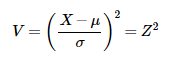is distributed as a chi-square random variable with 1 degree of freedom.

## 27. What is a Z test, Chi Square test, F test and T test?

Z-test is a statistical test where normal distribution is applied and is basically used for dealing with problems related to large samples when n (sample size) ≥ 30 .

It is used to determine whether two population means are different when the variances are known and the sample size is large. The test statistic is assumed to have a normal distribution and parameters such as standard deviation should be known in order for z-test to be performed.A one-sample location test, two-sample location test, paired difference test and maximum likelihood estimate are examples of tests that can be conducted as z-tests

Z-tests are closely related to t-tests, but t-tests are best performed when an experiment has a small sample size. Also, t-tests assume that the standard deviation is unknown, while z-tests assume that it is known. If the standard deviation of the population is unknown, the assumption that the sample variance equals the population variance is made.

It implements a z-test similar to the t.test function.

Usage:

simple.z.test(x, sigma, conf.level=0.95)

T-test assesses whether the means of two groups are statistically different from each other

A two-sample t-test examines whether two samples are different and is commonly used when the variances of two normal distributions are unknown and when an experiment uses a small sample size

For example, a t-test could be used to compare the average floor routine score of the U.S. women’s Olympic gymnastic team to the average floor routine score of China’s women’s teamIt performs one and two sample t-tests on vectors of data.

Usage:

t.test(x, …)

## Default S3 method:

t.test(x, y = NULL,

alternative = c(“two.sided”, “less”, “greater”),

mu = 0, paired = FALSE, var.equal = FALSE,

conf.level = 0.95, …)

## S3 method for class ‘formula’

t.test(formula, data, subset, na.action, …)

Chi square is a statistical test used to compare the observed data with the data that we would expect to obtain according to a specific hypothesis.

Formula for the chi square test is:

chisq.test performs chi-squared contingency table tests and goodness-of-fit tests.

Usage: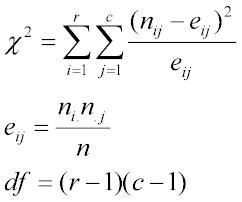chisq.test(x, y = NULL, correct = TRUE,

p = rep(1/length(x), length(x)), rescale.p = FALSE,

simulate.p.value = FALSE, B = 2000)

The F-test is designed to test if two population variances are equal. It does this by comparing the ratio of two variances. So, if the variances are equal, the ratio of the variances will be 1.Usage:

var.test(x, …)

## Default S3 method:

var.test(x, y, ratio = 1,

alternative = c(“two.sided”, “less”, “greater”),

conf.level = 0.95, …)

## S3 method for class ‘formula’

var.test(formula, data, subset, na.action, …)

I hope you enjoyed reading this blog. The need for Data Science with Python professionals has increased dramatically, making this course ideal for people at all levels of expertise. The Data Science Masters Program is ideal for professionals in analytics who are looking to work in conjunction with Python, Software, and IT professionals who are interested in the area of Analytics, and anyone with a passion for Data Science.

Related Posts:

10 Reasons Why Big Data Analytics is the Best Career Move

Upcoming Batches For Data Science with Python Certification Course
Course NameDate
Data Science with Python Certification Course

Class Starts on 2nd December,2023

2nd December

SAT&SUN (Weekend Batch)
View Details
Data Science with Python Certification Course

Class Starts on 3rd February,2024

3rd February

SAT&SUN (Weekend Batch)
View Details
•Rohit Chawhan says:

Usually I never comment on blogs but your article is so convincing that I never stop myself to say something about it. You’re doing a great job Man,Keep it up.
http://www.bisptrainings.com

•Balaji Sundar says:

Great list of questions and answers ! Very useful

•EdurekaSupport says:

Thanks so much, Balaji! We’re happy we could be of help. We urge you to keep checking our blog page for new blogs.REGISTER FOR FREE WEBINARThank you for registering Join Edureka Meetup community for 100+ Free Webinars each month

### Subscribe to our Newsletter, and get personalized recommendations.#### Top Data Science Interview Questions For Budding Data Scientists In 2024

edureka.co